# AP Chemistry : Endergonic and Exergonic Reactions

## Example Questions

### Example Question #1 : Endergonic And Exergonic Reactions

Which of these conditions will result in a spontaneous reaction?

Exothermic reactions with reactants going from liquid to gaseous state

All exothermic reactions

Exothermic reactions with reactants going from gaseous to liquid state

Endothermic reactions with reactants going from gaseous to liquid state

Endothermic reactions with reactants going from liquid to gaseous state

Exothermic reactions with reactants going from liquid to gaseous state

Explanation:

To determine whether a reaction will occur spontaneously, one needs to look at whether the Gibbs free energy will decrease. In order for Gibbs free energy to decrease, the combination of the heat of the reaction and the change in entropy must give a negative Gibbs free energy. The equation is: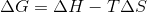If a reaction is exothermic, the heat of the reaction is negative. If the reactants go from liquid to gaseous state, the entropy increases (is positive). When the enthalpy is negative and the entropy is positive, the equation must always give a negative solution. This leads to a negative Gibbs free energy, so the reaction will be spontaneous.

### Example Question #11 : Thermodynamics

Which of the following reaction conditions cannot result in a spontaneous reaction?

Endothermic reactions in which reactants go from gaseous to liquid state

Exothermic reactions in which reactants go from liquid to solid state

Endothermic reactions in which reactants go from liquid to gaseous state

Exothermic reactions in which reactants go from gaseous to liquid state

Exothermic reactions in which reactants go from liquid to gaseous state

Endothermic reactions in which reactants go from gaseous to liquid state

Explanation:

To determine whether a reaction will occur spontaneously, one needs to look at whether the Gibbs free energy will decrease. In order for Gibbs free energy to decrease, the combination of the heat of the reaction and the change in entropy must give a negative Gibbs free energy. The equation is:If a reaction is endothermic, the heat of the reaction is positive. If the reactants go from gaseous to liquid state, the entropy decreases (is negative). When the enthalpy is positive and the entropy is negative, the equation must always give a positive solution. This leads to a positive Gibbs free energy, so the reaction can never be spontaneous.

### Example Question #3 : Endergonic And Exergonic Reactions

Which of the following values must be negative in order for a reaction to be spontaneous?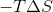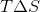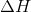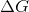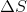In order to determine if a reaction is spontaneous, one needs to look at, which is the change in Gibbs free energy. The formula to find change in Gibbs free energy is:andcan have positive or negative values (under certain conditions), butmust always be negative in order for the reaction to be spontaneous.